Vertex Cover Problem

# Vertex Cover Problem - Theory of Computation - Computer Science Engineering (CSE)

Part VI. Vertex Cover Problem

10 Vertex Cover Problem

Vertex Cover
A vertex cover of a graph is a set of vertices, V such that if (a, b) is an edge of a graph, then a or b or both must be present in V.
Consider the following graph: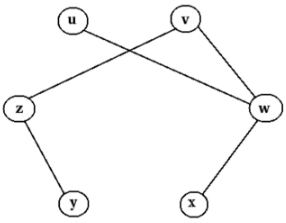In the above graph, vertex cover is {z, w}.
Let V= {z, w}
The edges of the above graph are uw, vw, xw, vz, yz. Note that in every edge, at least one vertex is a member of V.
For example,
Consider the edge uw. Here, vertex w ∈ V.
Consider the edge vz. Here, vertex z ∈ V.
Thus, {z, w} form a vertex cover.

Vertex Cover Problem
The vertex cover problem is to check whether a graph has a vertex cover of size k.

Vertex Cover Problem is NP-Complete
We know that a problem is in class NP-Complete if: it is in NP and it is NP Hard.

Theorem:
Vertex cover problem is NP-complete.

Proof:

Step 1: Prove that vertex cover problem is in class NP.
A set with n elements has 2n possible subsets. Then a graph with n vertices has 2n possible subsets of vertices. So an algorithm needs to check whether any one of these subsets form a vertex cover. It has worst case time complexity Θ(2n). (not polynomial time, but exponential time). Let we are given a graph and a ’certificate’ telling that a subset of vertices form a vertex cover. An algorithm can verify whether at leat one vertex of every edge in the graph is an element of this vertex cover in polynomial time.
So vertex cover problem is in class NP.

Step 2: Prove that vertex cover problem is NP-hard.
We know that a problem is NP-Hard, if every problem in NP can be reduced to this problem in polynomial time. The approach we used is as follows: In the previous section, we already proved that all NP problems can be reduced to SAT. Then, again we found that SAT problem can be reduced to 3-CNF-SAT problem. Again, we reduced 3-CNF-SAT problem to clique problem. Then, if we can reduce clique problem to vertex cover problem in polynomial time, we can say that vertex cover problem is NP-hard.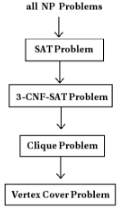We need to reduce clique problem to vertex cover problem.
Consider the following graph with clique {u, v, x, y}.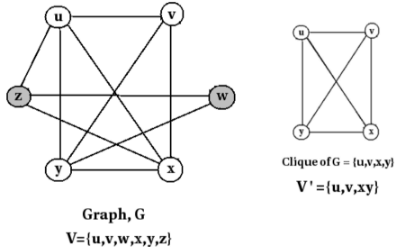Take the complement of the above graph, G. That is G.G is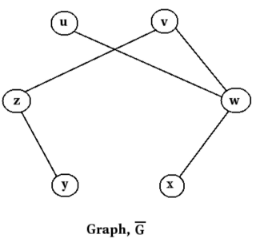For the graph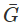vertex cover is {z,w}.
This vertex cover is found by V - V’.
That is, {u,v,w,x,y,z} - {u,v,x,y} = {z,w}
So from the clique, we can find out the vertex cover of a graph using the above mechanism.

Thus the given clique problem is reduced to vertex cover problem. This means vertex cover problem is NP-hard.

In step 1, we proved that vertex cover problem is in NP.
In step 2, we proved that vertex cover problem is NP-hard.
So, vertex cover problem is an NP-complete problem.

The document Vertex Cover Problem | Theory of Computation - Computer Science Engineering (CSE) is a part of the Computer Science Engineering (CSE) Course Theory of Computation.
All you need of Computer Science Engineering (CSE) at this link: Computer Science Engineering (CSE)

## Theory of Computation

18 videos|56 docs|44 tests

## FAQs on Vertex Cover Problem - Theory of Computation - Computer Science Engineering (CSE)

 1. What is the Vertex Cover Problem in Computer Science Engineering (CSE)?Ans. The Vertex Cover Problem in CSE is a well-known computational problem that involves finding the minimum number of vertices needed to cover all edges in a given graph. It is a critical problem in various areas, including network design, scheduling, and optimization.
 2. How is the Vertex Cover Problem relevant to Computer Science Engineering (CSE) exams?Ans. The Vertex Cover Problem is often included in CSE exams as it tests students' understanding of graph theory, algorithms, and problem-solving skills. It helps evaluate their ability to analyze and solve real-world problems using computational techniques.
 3. What are the applications of the Vertex Cover Problem in CSE?Ans. The Vertex Cover Problem has several practical applications in CSE. It is used in network design to minimize the number of network nodes required to cover all connections, in scheduling problems to optimize resource allocation, and in database management systems to enhance query performance by selecting the most relevant data.
 4. What are the common algorithms used to solve the Vertex Cover Problem in CSE?Ans. Various algorithms are employed to solve the Vertex Cover Problem in CSE. Some popular ones include the brute-force algorithm, which exhaustively checks all possible vertex combinations, and approximation algorithms like the Greedy algorithm and the 2-Approximation algorithm that provide efficient and near-optimal solutions.
 5. Can the Vertex Cover Problem be solved in polynomial time?Ans. The Vertex Cover Problem is a known NP-complete problem, which means that no polynomial-time algorithm has been discovered yet to solve it optimally. However, there are efficient approximation algorithms that provide close-to-optimal solutions in polynomial time, making them practical for solving large-scale instances of the problem.

## Theory of Computation

18 videos|56 docs|44 testsExplore Courses for Computer Science Engineering (CSE) examSignup to see your scores go up within 7 days! Learn & Practice with 1000+ FREE Notes, Videos & Tests.
10M+ students study on EduRev
Track your progress, build streaks, highlight & save important lessons and more!
Related Searches

,

,

,

,

,

,

,

,

,

,

,

,

,

,

,

,

,

,

,

,

,

;